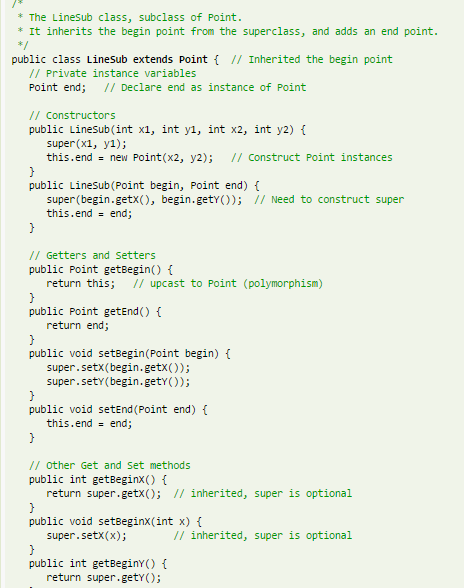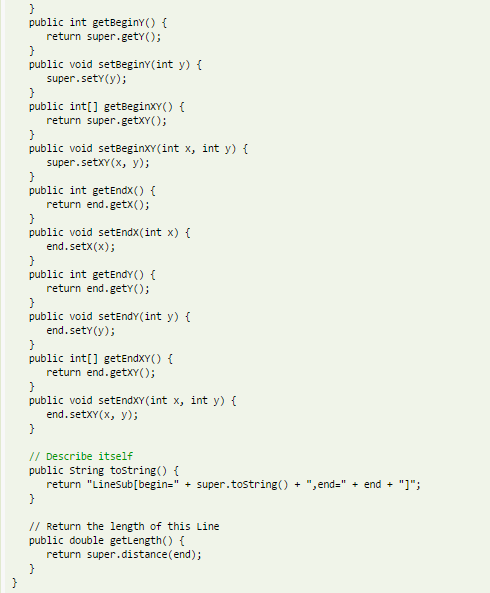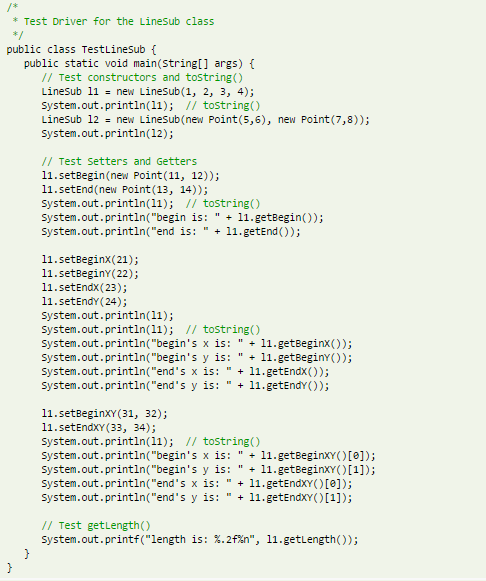# (Solved Homework): I am trying to plot a function in python 2.7.12 and keep getting a syntax error at xd3.append(0). I'm basing the script

I am trying to plot a function in python 2.7.12 and keep getting a syntax error at xd3.append(0). I’m basing the script off of another script through which my function was derived, which I will attach as well. Can someone help me revise my script so that I can actually plot it out? Thanks so much!

My Script:

import math
from numpy import matrix
from numpy import linalg
import numpy
import matplotlib.pyplot as plt
import sys
m = 11
first = 0
second = 0
pi = math.pi

# delare an empty list to hold values of a
a = []

# getting input from user
for i in range(0,m):
a.append( float(input(‘Enter a value of a[‘ + str(i) + ‘]: ‘)) )

# calculation for summation
# in function range(1,m), 1 is included and m is excluded. So effectively we go from 1 to m-1.
first = 0.0
second = 0.0
for j in range(1, m):
temporary_sum_first = 0.0
temporary_sum_second = 0.0
for i in range(0, m):
# this is the function I want to plot
temporary_sum_first += (a[i] * math.cos(2*pi*i*j/m))
temporary_sum_second += ((-a[i])*i* math.sin(2*pi*i*j/m))

# now square it and sum it
first = first + math.pow(temporary_sum_first, 2)
second = second + math.pow(temporary_sum_second, 2)
# at this point, we have finished summing i=0 to i=m-1 for one value of p, we continue this for m-1 values of j.

#Final Summation Value
Fmin=first+second
print(“Summation value:”)
print(Fmin)
#If you comment out/ delete everything after this point, you can sub in values for ai
# and find the value of the minimization function
#Now I want to plot out what it looks like
def f(theta,x,m):

for j in range(1, m):
temporary_sum_first = 0.0
temporary_sum_second = 0.0
#This is where I think I went wrong somehow
fsum = temportary_sum_first + temporary_sum_second
for i in range(0, m):
temporary_sum_first += (a[i] * math.cos(2*pi*i*j/m))
temporary_sum_second += ((-a[i])*i* math.sin(2*pi*i*j/m))
return fsum

mhalf = m/2
offt = pi/8
t_min = – offt
t_max = 2*pi +offt
Npts = 500
dt = (t_max – t_min)/Npts

Xv = []
Yv = []
Zv = []
for i in range(Npts):
Xv.append(t_min + dt*i)
Yv.append(f(Xv[i],x,m))
Zv.append(0.0)

Xd = []
Yd = []
Xd2 = []
Yd2 = []
Xd3 = []
Yd3 = []
for i in range(mhalf):
Xd.append(2*(i+1)*pi/m)
Yd.append(0)
Xd2.append(2*(i+mhalf +1)*pi/m
Xd3.append(0)
Xd3.append(2*pi)
Yd3.append(1)
Yd3.append(1)

p1 = plt.subplot(111)
p1.plot(Xv, Yv, color=’red’, lw=2)
p1.plot(Xv, Zv, color=’blue’, lw=2)
p1.scatter(Xd, Yd, color=’black’, marker=’o’, facecolors=’none’, edgecolors=’k’, s=200, lw=2)
p1.scatter(Xd2, Yd2, color=’yellow’, marker=’o’, facecolors=’none’, edgecolors=’y’, s=200, lw=2)
p1.scatter(Xd3, Yd3, color=’black’, marker=’o’, s=200, lw=2)
p1.set_xlim([t_min,t_max])
p1.set_xlabel(r’\$theta\$’,size=28)
p1.set_ylabel(r’\$f(theta)\$’,size=28)
title = r”\$m = %2.0f\$” % m
p1.set_title(title,fontsize=32,color=’black’)

figFile = ‘fig_’+str(m)+’_0.eps’
#plt.savefig(figFile,format=’eps’)
plt.show()

Reference Script:

from numpy import matrix
from numpy import linalg
import numpy
import matplotlib.pyplot as plt
from math import *
import sys

def f(theta,x,Nd):
fsum = 0.0
for i in range(Nd):
fsum = fsum + x[i]*cos(i*theta)
return fsum
Nd=11
#Nd = int(sys.argv) # Command-line arg: kicking frequency
Ndhalf = Nd/2
offt = pi/8
t_min = -offt
t_max = 2*pi + offt
Npts = 500
dt = (t_max – t_min)/Npts

# Solution
x = []
x.append(1.0/Nd)
for i in range(Nd-1):
x.append(2*(Nd-i-1)/(Nd**2.0))
print i, x[i]

Xv = []
Yv = []
Zv = []
for i in range(Npts):
Xv.append(t_min + dt*i)
Yv.append(f(Xv[i],x,Nd))
Zv.append(0.0)

Xd = []
Yd = []
Xd2 = []
Yd2 = []
Xd3 = []
Yd3 = []
for i in range(Ndhalf):
Xd.append(2*(i+1)*pi/Nd)
Yd.append(0)
Xd2.append(2*(i+Ndhalf+1)*pi/Nd)
Yd2.append(0)
Xd3.append(0)
Xd3.append(2*pi)
Yd3.append(1)
Yd3.append(1)

p1 = plt.subplot(111)
p1.plot(Xv, Yv, color=’red’, lw=2)
p1.plot(Xv, Zv, color=’blue’, lw=2)
p1.scatter(Xd, Yd, color=’black’, marker=’o’, facecolors=’none’, edgecolors=’k’, s=200, lw=2)
p1.scatter(Xd2, Yd2, color=’yellow’, marker=’o’, facecolors=’none’, edgecolors=’y’, s=200, lw=2)
p1.scatter(Xd3, Yd3, color=’black’, marker=’o’, s=200, lw=2)
p1.set_xlim([t_min,t_max])
p1.set_xlabel(r’\$theta\$’,size=28)
p1.set_ylabel(r’\$f(theta)\$’,size=28)
title = r”\$m = %2.0f\$” % Nd
p1.set_title(title,fontsize=32,color=’black’)

figFile = ‘fig_’+str(Nd)+’_0.eps’
#plt.savefig(figFile,format=’eps’)
plt.show()Order NOW for a 10% Discount
Pages (550 words)
Approximate price: -

Why Us?Top Quality and Well-Researched Papers

All ourbpapers are written from scratch. In fact, Clients who ask for paraphrasing services are highly discouraged. We have writers ready to craft any paper from scratch and deliver quality ahead of time.Our writers keeps you posted on your papers progress - providing you with paper outline/draft. You are also at liberty to communicate directly with your writer.Free Unlimited Revisions

If you think we missed something, send your order for a free revision. You have 10 days to submit the order for review after you have received the final document. You can do this yourself after logging into your personal account or by contacting our support.Prompt Delivery and 100% Money-Back-Guarantee

All papers are always delivered on time, in many cases quite ahead of time. In case we need more time to master your paper, we may contact you regarding the deadline extension. In case you cannot provide us with more time, a 100% refund is guaranteed.Original & Confidential

We use several writing tools checks to ensure that all documents you receive are free from plagiarism. Our editors carefully review all quotations in the text. We also promise maximum confidentiality in all of our services.Our support agents are available 24 hours a day 7 days a week and committed to providing you with the best customer experience. Get in touch whenever you need any assistance.

Try it now!

## Calculate the price of your order

Total price:
\$0.00

How it works?Fill in the order form and provide all details of your assignment.Proceed with the payment

Choose the payment system that suits you most.Our Services

No need to work on your paper at night. Sleep tight, we will cover your back. We offer all kinds of writing services.## Essay Writing Service

No matter what kind of academic paper you need and how urgent you need it, you are welcome to choose your academic level and the type of your paper at an affordable price. We take care of all your paper needs and give a 24/7 customer care support system.An admission essay is an essay or other written statement by a candidate, often a potential student enrolling in a college, university, or graduate school. You can be rest assurred that through our service we will write the best admission essay for you.Editing Support

Our academic writers and editors make the necessary changes to your paper so that it is polished. We also format your document by correctly quoting the sources and creating reference lists in the formats APA, Harvard, MLA, Chicago / Turabian.Revision Support

If you think your paper could be improved, you can request a review. In this case, your paper will be checked by the writer or assigned to an editor. You can use this option as many times as you see fit. This is free because we want you to be completely satisfied with the service offered.